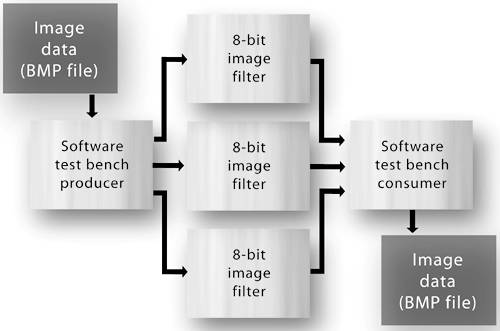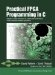# Section 11.3. Refinement 1: Creating Parallel 8-Bit Filters

### 11.3. Refinement 1: Creating Parallel 8-Bit Filters

As written, the inner code loop of this edge-detection process requires a total of 18 instruction stages (six stages per color), which may or may not be fast enough to meet the requirements. We can attempt to speed up this process using the techniques described in Chapter 9, but these optimizations are unlikely to accelerate the loop dramatically due to the number of memory accesses being performed on the bytebuffer array.

In particular, consider the following statements at the beginning and end of the inner code loop:

` while ( co_stream_read(pixels_in, &nPixel, sizeof(co_uint24)) == co_err_none ) {   bytebuffer[addpos][REDINDEX] = nPixel & REDMASK;   bytebuffer[addpos][GREENINDEX] = (nPixel & GREENMASK) >> 8;   bytebuffer[addpos][BLUEINDEX] = (nPixel & BLUEMASK) >> 16; `

These statements unpack the 24-bit value obtained from the stream to create three distinct references to the red, green, and blue pixel values, consuming three clock cycles in doing so. After unpacking, the edge detection is performed for each of the three colors in the inner code loop, as summarized here again:

` for (clr = 0; clr < 3; clr++) {  // Red, Green and Blue    pixelN  = bytebuffer[B_OFFSETADD(currentpos,WIDTH)][clr];    pixelS  = bytebuffer[B_OFFSETSUB(currentpos,WIDTH)][clr];    . . . }  `

One approach to accelerating this algorithm might be to perform these three edge-detection operations in parallel, by creating three instances of the same image filter. This should, in theory, reduce the amount of time needed to process one pixel by a factor of three, plus whatever overhead is required to pack and unpack the 24-bit pixel values. In this application, which reads its inputs from an RGB-encoded source file, the pixels are already available as distinct color values. Therefore, the test bench (the C function that streams pixel data to the edge-detection process for the purpose of testing) can be easily modified to generate three streams of pixel color values, rather than one stream of whole pixel values, as in the original version.

As an initial attempt to speed up this edge-detection filter, we will create parallelism at the algorithm level by partitioning the algorithm into three distinct edge detection filters, one for each color, as illustrated in Figure 11-5.

##### Figure 11-5. Creating three parallel instances of an 8-bit edge-detection process.The resulting eight-bit, one-color filter (which is replicated three times) is shown in Figure 11-6. In this listing, notice that

• The input stream (pixels_in) is now used to transfer unsigned eight-bit values, as specified in the co_stream_open function.

• As in the original version, the bytebuffer array is used to store pixel color values. In the single-color version, bytebuffer is only a one-dimensional array.

##### Figure 11-6. Eight-bit edge-detection process.
` void edge_detect(co_stream pixels_in, co_stream pixels_out) {   int currentpos, addpos, idx = 0;   short pixeldiff1, pixeldiff2, pixeldiff3, pixeldiff4;   short pixelN, pixelS, pixelE, pixelW, pixelNE, pixelNW, pixelSE, pixelSW;   co_uint8 pixelMag;   co_uint8 bytebuffer[BYTEBUFFERSIZE];   co_stream_open(pixels_in, O_RDONLY, UINT_TYPE(8));   co_stream_open(pixels_out, O_WRONLY, UINT_TYPE(8));   // Begin by filling the bytebuffer array...   idx = 0;   while (co_stream_read(pixels_in,&bytebuffer[idx],sizeof(co_uint8))                                                  == co_err_none ) {     idx++;     if (idx == BYTEBUFFERSIZE - 1)         break;   }   // Now we have an almost full buffer and can start processing pixel   // windows. But first, we need to write out the first line of pixels and   // the first pixel of the second line (they don't get processed):   for(idx=0; idx < WIDTH+1; idx++) {      co_stream_write(pixels_out, &bytebuffer[idx], sizeof(co_uint8));   }    // Now, each time we process a window we will "shift" the buffers   // one position and read in a new pixel. We will continue this until   // the input stream is closed (eos). "Shifting" is accomplished by   // manipulating the currentpos and addpos index variables.   addpos = BYTEBUFFERSIZE - 1;   currentpos = WIDTH;   // Read pixel values from the stream...   while ( co_stream_read(pixels_in, &bytebuffer[addpos],            sizeof(co_uint8)) == co_err_none ) { #pragma CO PIPELINE     addpos++;     if (addpos == BYTEBUFFERSIZE)         addpos = 0;     currentpos++;     if (currentpos == BYTEBUFFERSIZE)         currentpos = 0;         // At this point we are guaranteed to have enough pixels in         // our array to process a window, so let's do it...         pixelN  = bytebuffer[B_OFFSETADD(currentpos,WIDTH)];         pixelS  = bytebuffer[B_OFFSETSUB(currentpos,WIDTH)];         pixelE  = bytebuffer[B_OFFSETADD(currentpos,1)];         pixelW  = bytebuffer[B_OFFSETSUB(currentpos,1)];         pixelNE = bytebuffer[B_OFFSETADD(currentpos,WIDTH+1)];         pixelNW = bytebuffer[B_OFFSETADD(currentpos,WIDTH-1)];         pixelSE = bytebuffer[B_OFFSETSUB(currentpos,WIDTH-1)];         pixelSW = bytebuffer[B_OFFSETSUB(currentpos,WIDTH+1)];         // Diagonal difference, lower right to upper left         pixeldiff1 = ABS(pixelSE - pixelNW);         // Diagonal difference, upper right to lower left         pixeldiff2 = ABS(pixelNE - pixelSW);         // Vertical difference, bottom to top         pixeldiff3 = ABS(pixelS - pixelN);         // Horizontal difference, right to left         pixeldiff4 = ABS(pixelE - pixelW);         pixelMag = (co_uint8) MAX4(pixeldiff1,pixeldiff2,pixeldiff3,pixeldiff4);         if (pixelMag < EDGE_THRESHOLD) {              pixelMag = 0;         }          co_stream_write(pixels_out, &pixelMag, sizeof(co_uint8));     }     // Write out the last line of the image (plus one extra pixel     // representing the last pixel on the end of the second to last     // line.)     for(idx = 0; idx < WIDTH+1; idx++) {          co_stream_write(pixels_out, &bytebuffer[currentpos], sizeof(co_uint8));         currentpos++;         if (currentpos > BYTEBUFFERSIZE)             currentpos = 0;     }     co_stream_close(pixels_in);     co_stream_close(pixels_out); } `

• Because we no longer require the innermost code loop, which previously processed each color in turn, we can now use the PIPELINE pragma. This optimization will improve this loop's throughout rate by two clock cycles but is of limited benefit due to the manner in which bytebuffer is accessed. As before, memory access continues to be a limiting factor in increasing the speed of this algorithm.

To complete this version of the application, we will need to create and interconnect three instances of the single-color filter process. The configuration function that describes this structure is shown in Figure 11-7. In this configuration function (which also refers to the software test bench function) we can see declarations for six streamsthree colors for the input side and three colors for the output sideas well as declarations and corresponding calls to co_process_create for the five processes: the three edge-detection processes, plus the producer and consumer test processes.

This version of the edge-detection algorithm will run substantially faster than the original version but will of course require more hardware when synthesized.Practical FPGA Programming in C
ISBN: 0131543180
EAN: 2147483647
Year: 2005
Pages: 208

Similar book on Amazon